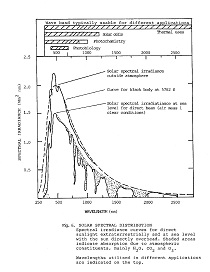# STATISTICS BSC303Learn to use statistics for a better understanding of business planning and management.

This course assumes a basic knowledge of Statistics (eg. high school level).
A 100 hour course such as this will not make you into a statistician, but it will provide the most essential knowledge and skills required by consultants and researchers in a wide variety of disciplines taught by ACS.

### Learn Statistics at Home

Statistics are scientific methods employed to gather, organise and analyse data. We are able to draw conclusions and make inferences on the basis of such analyses. Descriptive statistics describe a set of data, while inferential statistics make inferences about large groups based on data from a smaller subset of the group. To infer means to draw a conclusion based on facts or premises. Thus an inference is the end result; a proposition based on the act of inferring.

### COURSE STRUCTURE

There are 10 lessons as follows:
1. Introduction
2. Distributions
3. Measures of central tendency
4. The Normal curve and Percentiles and Standard Scores
5. Correlation
6. Regression
7. Inferential Statistics
8. The t Test
9. Analysis of variance
10. Chi square test

Each lesson culminates in an assignment which is submitted to the school, marked by the school's tutors and returned to you with any relevant suggestions, comments, and if necessary, extra reading.

#### Aims

• Become familiar with different statistical terms and the elementary representation of statistical data.
• Become familiar with distributions, and the application of distributions in processing data.
• Apply measures of central tendency in solving research questions
• Demonstrate and explain the normal curve, percentiles and standard scores.
• Explain methods of correlation that describes the relationship between two variables.
• Make predictions with regression equations.
• Determine how much error to expect when making the predictions.
• Explain the basic concepts of underlying the use of statistics to make inferences.
• Analyze the difference between the means of two groups with the t Test.
• Describe the use of ANOVA (Analysis of Variance) in analysing the difference between two or more groups.
• Apply the concept of Non Parametric Statistics.

In summary statistics are useful in quantifying characteristics of a population based on samples from that population. An effective experiment design will yield useful reliable statistics. Measurements can be made and data presented in different ways to facilitate visualising the data

#### Do You Understand Correlation?

A very important part of statistics is describing the relationship between two (or more) variables. One of the most fundamental concepts in research is the concept of correlation. If two variables are correlated, this means that you can use information about one variable to predict the values of the other variable.   Correlation is used to denote the direction and strength of a linear relationship.

Despite the apparently ever-changing view of the environment, when you look a little deeper, there are some things that do not change that much or change in a systematic and predictable way (sometimes referred to as invariant relationships). In some areas of science we can predict things quite accurately indeed. The movements of the planets, and the moons around the planets, can be predicted quite accurately centuries into the future from our current knowledge of orbits and physics. In the behavioural sciences things are not quite as predictable. We can only talk about an increased likelihood or a decreased likelihood of something happening. We try to make predictions in a much more uncertain environment. But we can still discover relationships and predict an increased likelihood or a decreased likelihood of something happening, given that something else has happened.

If we were to gather data on hair colour and income, we would not expect to find that hair colour had an influence on income.  Most likely we would not be able to find any relationship at all, regardless of how hard we looked.  We would say that there is zero correlation between the two variables (hair colour and income).

However if we found that every time we increased the processing speed of the chip in our computer, the speed of our computer increased proportionally, we would have found a perfect positive correlation (+1).

We can also have a perfect negative correlation (-1).  For example, if we tested a class of students at mathematics and found that the ranking of each student was reversed for sport we would have found a perfect negative correlation.

#### Statistics Helps Us Manage Business Better

The performance of a business, good or bad, is judged using statistics; and without a knowledge of statistics, it can be difficult to understand what is happening in your business. Statistics are used to indicate trends, and what is likely to change and how fast it is likely to change. Business managers can use statistics to determine where profits are likely to be better; where  potential is for growth, and where business is in decline. There are lots of things we can learn about a business though statistics; not only where it has been but where it is likely to be in the future.

If you have not studied statistics before; and are wanting to be more successful at running a business in the future; this course can be very useful.